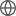Old Web
English
Luminous $z\ge7$ quasars provide direct probes of the evolution of supermassive black holes (SMBHs) and the intergalactic medium (IGM) during the epoch of reionization (EoR). The Ly$\alpha$ damping wing absorption imprinted by neutral hydrogen in the IGM can be detected in a single EoR quasar spectrum, allowing the measurement of the IGM neutral fraction towards that line of sight. However, damping wing features have only been detected in two $z>7$ quasars in previous studies. In this paper, we present new high quality optical and near-infrared spectroscopy of the $z=7.00$ quasar DES J025216.64--050331.8 obtained with Keck/NIRES and Gemini/GMOS. By using the MgII single-epoch virial method, we find that it hosts a $\rm (1.39\pm0.16) \times10^{9} ~M_\odot$ SMBH accreting at an Eddington ratio of $\lambda_{\rm Edd}=0.7\pm0.1$, consistent with the values seen in other luminous $z\sim 7$ quasars. Furthermore, the Ly$\alpha$ region of the spectrum exhibits a strong damping wing absorption feature. The lack of associated metal absorption in the quasar spectrum indicates that this absorption is imprinted by a neutral IGM. Using a state-of-the-art model developed by Davies et al., we measure a volume-averaged neutral hydrogen fraction at $z=7$ of $\langle x_{\rm HI} \rangle = 0.70^{+0.20}_{-0.23} (^{+0.28}_{-0.48})$ within 68% (95%) confidence intervals when marginalizing over quasar lifetimes of $10^3\le t_{\rm Q}\le10^8$ yr. This is the highest IGM neutral fraction yet measured using reionization-era quasar spectra.